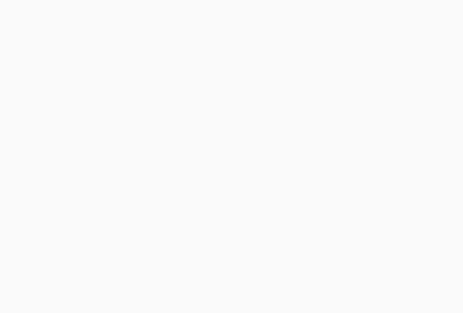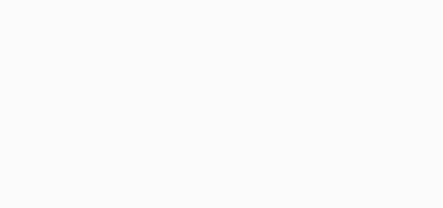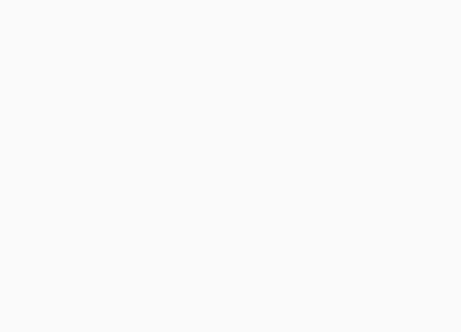#Aptitude Questions: Data Interpretation Set 67

Hello Aspirants.

Welcome to Online Quantitative Aptitude section in AffairsCloud.com. Here we are creating question sample From Data Interpretation that is important for all the competitive exams. We have included Some questions that are repeatedly asked in exams.

I.Study the following information carefully to answer the given Questions

The pie chart gives the market share of laptop sellers and line chart gives the saes of Lenovo for 2012 -13 to 2016-171. What is the percentage increase in the sales of Lenovo from 2012 -13 to 2015 -16 ?
1.65.72%
2.90.50%
3.76.90%
4.88.89%
5.None of these
Explanation :
2012-13 = 90
2015-16 = 170
% = 170-90/90 * 100 = 88.89%

2. If the sales of Acer laptop in 2015-16 is equal to 36% of Lenovo laptop Share in 2014-15 and Lenovo market share of 2014-15 is same as 2015-16, then what is the sales of acer laptop in 2015-16 ?
1.60.5cr
2.72cr
3.57.25cr
4.45.80cr
5.None of these
Explanation :
Acer in 2015-16 =  36*45/100 = 16.2 = 16%
Lenovo = 45% = 170
100% = 170*100/ 45 = 377.7 = 378
Acer = 378*16/100 = 60.5 crore

3. If the total sales of Compaq in 2014 -15 is Rs.25.75cr. The % of sales is decreased by 2.7% in 2015-16, then find the total sales of Compaq in 2015-16 ?
1.22.75cr
2.25.05cr
3.26 cr
4.23.57cr
5.None of these
Explanation :
7% = 25.75cr
2.7% decrease = 25.75*97.3/100 = 25.05 cr

4. What is the average sales of  Lenovo laptop from 2012 – 13 to 2015-16 ?
1.125. 5 cr
2.132.7 cr
3.127.5 cr
4.117.5 cr
5.None of these
Explanation :
Total = 90+112+138+170 = 510
Avr = 510/4 = 127.5 cr

5. If HCL Laptop Price is Rs.40,000 in 2014-15 is.Find the no of HCL laptop sold in 2014-15 ?
1.9200
2.8500
3.10200
4.7600
5.None of these
Explanation :
45% = 138
100% = 138*100/45 = 306.7 cr
12% = 306.7*12/100 = 36.8 cr
No of Laptop = 36,80,00,000/40,000 = 36800/4 = 9200

II.Study the following information carefully to answer the given Questions1. If the sales of Himalaya increased by 10% per year and if the sales of Fair & lovely is Rs.250 cr in 2013, what will be the sales of Himalaya in 2015 ?
1.260.5 cr
2.242.3 cr
3.254.1 cr
4.252 cr
5.None of these
Explanation :
25% = 250cr
100% = 250*100/25 = 1000 cr
Himalays 21% = 1000*21/100 = 210 cr
10% increase every year = 210*110/100 * 110/100 = 254.1 cr

2. What will be the central angle of Amway, Ponds and lakme together ?
1.161.2o
2.152.5o
3.150.1o
4.151.2o
5.None of these
Explanation :
Total = 19+8+15 = 42
Angle = 42*360/100 = 151.2o

3. If the total sales of cosmetic product in the market is Rs.670cr/ what is the difference between the sales of Lakme and Dove ?
1.17.5 Cr
2.20.1 Cr
3.25.6 Cr
4.32. 7 Cr
5.None of these
Explanation :
100% = 670cr
Lakme = 15*670/100 =100.5 Cr
Dove = 12*670/100 = 80.4 Cr
Diff = 100.5 – 80.4 = 20.1 Cr

4. If the cosmetic have only 12% share of the total market products, what is the share of the Himalaya in the total market(in %) ?
1.2.52%
2.3.15%
3.4%
4.1.75%
5.None of these
Explanation :
Himalaya = 21%
Total Market share = 12%
Himalaya in Total Market = 21*12/100 = 2.52%

5. If the sales of Fair & Lovely is estimated as Rs.20.8cr, Find the sales of Ponds(in lakhs) ?
1.Rs.6,05,60,000
2.Rs.6,65,00,000
3.Rs.6,65,000
4.Rs.6,65,60,000
5.None of these